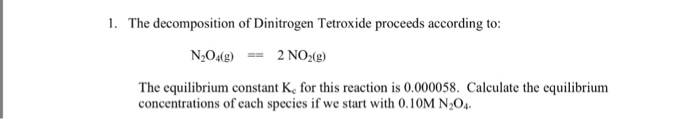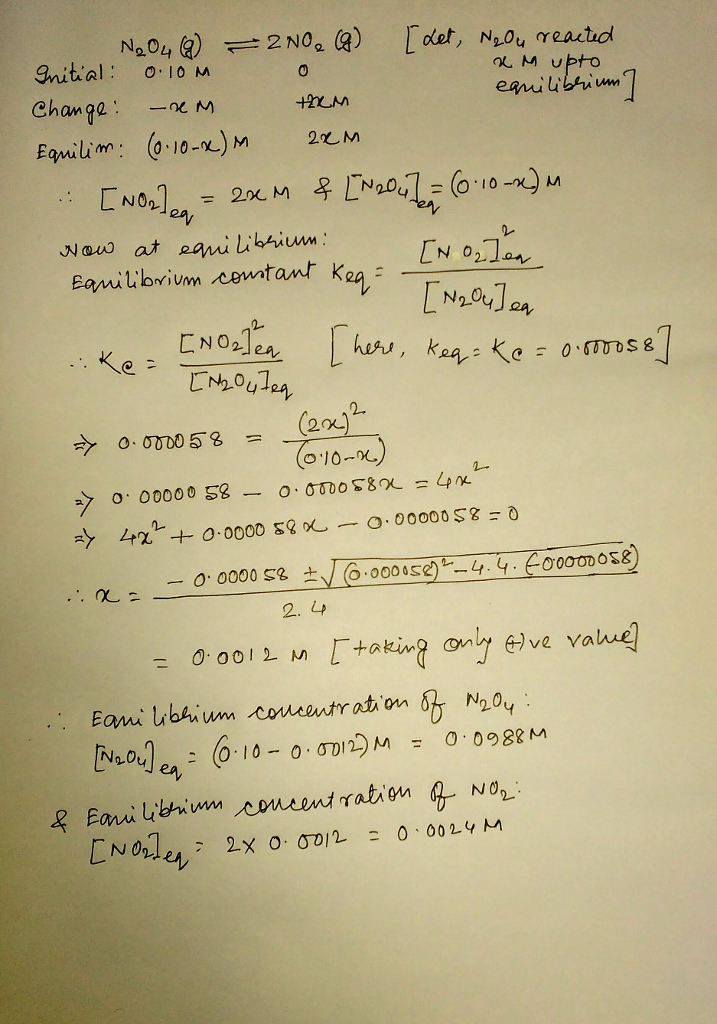# Question & Answer: The decomposition of Dinitrogen Tetroxide proceeds according to: N_2O_4(g) == 2NO_2(g)…..The decomposition of Dinitrogen Tetroxide proceeds according to: N_2O_4(g) == 2NO_2(g) The equilibrium constant K_c for this reaction is 0.000058. Calculate the equilibrium concentrations of each species if we start with 0.10M N_2O_4.